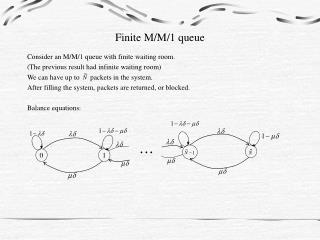# Finite M/M/1 queue - PowerPoint PPT PresentationDownload PresentationFinite M/M/1 queue

Finite M/M/1 queue
Download Presentation## Finite M/M/1 queue

- - - - - - - - - - - - - - - - - - - - - - - - - - - E N D - - - - - - - - - - - - - - - - - - - - - - - - - - -
##### Presentation Transcript

1. Finite M/M/1 queue Consider an M/M/1 queue with finite waiting room. (The previous result had infinite waiting room) We can have up to packets in the system. After filling the system, packets are returned, or blocked. Balance equations: 0 1

2. We have a steady state distribution for all . Of particular interest is

3. system Consider any queue with blocking probability PB and load  packets/second. Net arrival rate = (1- PB) . Then  = (1- PB)  = throughput. From a different point of view,

4. For M/M/1 queue of finite length,

5. M/M/m Queue There are m servers and the customers line up in one queue. The customer at the head of the queue is routed to the available server. Balance equations: 0 1 ………. … m+1 m+2 m-1 m …

6. Erlang C Formula

7. M/M/ Queue

8. M/M/m/m/ Queue There are m servers. If a customer upon arrival finds all servers busy, it does not enter the system and is lost. The m in •/•/•/m is the limit of the number of customers in the system. This model is used frequently in the traditional telephony. To use in the data networks, we can assume that m is the number of virtual circuit connections allowed. Balance equations: …. m-1 m 0 1

9. Multi-Dimensional Markov Chain Consider transmission lines with m independent circuits of equal capacity. There are two types of sessions:

10. Transition Probability Diagram m, 0 m-1, 0 m-1, 1 . . . . . . . . . 1, m-1 1,0 1,2 1,1 . . . 0, m-1 0, m 0,2 0,1 0,0

11. k,0 k,1 k, m-k …. Suppose in the previous case, there is a limit k < m on the number of circuits that can be used by sessions of type 2. k-1, 2 k, m-k k-1,0 k-1, 1 …. k-1, m-k-1 …. …. …. …. …. 1, m-1 1,0 …. 1,2 1,1 0, m 0, m-1 …. 0,2 0,1 0,0 Blocking probabilities for call types:

12. Method of fining the steady state solutions for multi dimensional Markov Chain.

13. Truncation of Multi Dimensional System Consider l M/M/1 in independent queues. Then, for the joint queue, the following is true: Above is also true for M/M/m, M/M/, M/M/m/m, and all other birth-death processes. We now consider truncation of multi dimensional Markov Chain. Truncation is achieved by eliminating (or not considering) some of the states with low probability. The truncated system is a Markov Chain with the same transition diagram without some of the states that have been eliminated.

14. Claim: Stationary distribution of the truncated system is in a product form. Proof: We have detailed balance equations: Substituting we can show that balance equations hold true with Since the solution satisfies the balance equations, it must the unique stationary distribution.

15. Example: Blocking Prob of Two Call Types

16. Important Results for M/G/1 Queue Pollaczek=Khinchin Formula: